#### WATCH ALL SLIDES

Slide 12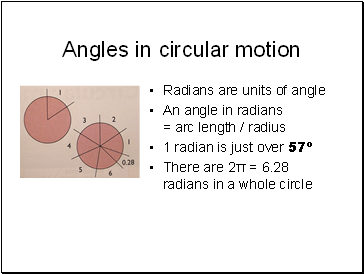## Angles in circular motion

1 radian is just over 57º

There are 2π = 6.28 radians in a whole circle

Slide 13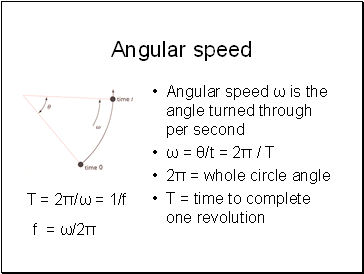## Angular speed

Angular speed ω is the angle turned through per second

ω = θ/t = 2π / T

2π = whole circle angle

T = time to complete one revolution

T = 2π/ω = 1/f

f = ω/2π

Slide 14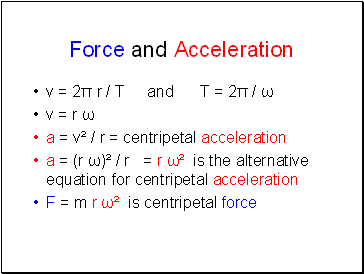## Force and Acceleration

v = 2π r / T and T = 2π / ω

v = r ω

a = v² / r = centripetal acceleration

a = (r ω)² / r = r ω² is the alternative equation for centripetal acceleration

F = m r ω² is centripetal force

Slide 15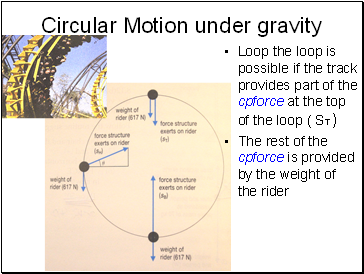## Circular Motion under gravity

Loop the loop is possible if the track provides part of the cpforce at the top of the loop ( ST )

The rest of the cpforce is provided by the weight of the rider

Slide 16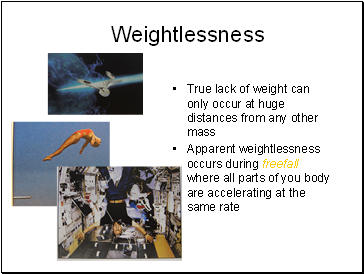## Weightlessness

True lack of weight can only occur at huge distances from any other mass

Apparent weightlessness occurs during freefall where all parts of you body are accelerating at the same rate

Slide 17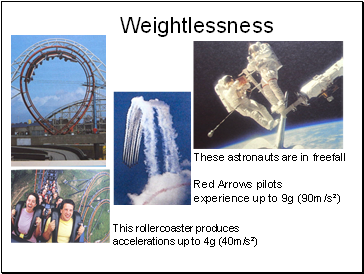## Weightlessness

This rollercoaster produces accelerations up to 4g (40m/s²)

These astronauts are in freefall

Red Arrows pilots experience up to 9g (90m/s²)

Slide 18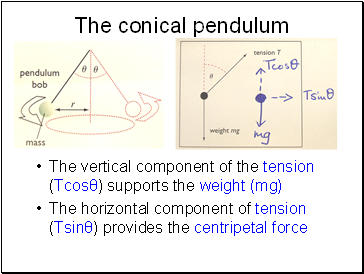## The conical pendulum

The vertical component of the tension (Tcosθ) supports the weight (mg)

The horizontal component of tension (Tsinθ) provides the centripetal force

Slide 19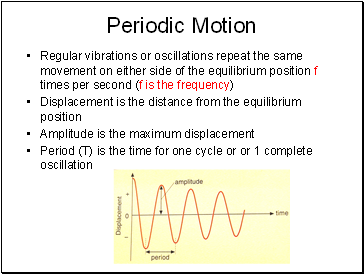## Periodic Motion

Regular vibrations or oscillations repeat the same movement on either side of the equilibrium position f times per second (f is the frequency)

Displacement is the distance from the equilibrium position

Amplitude is the maximum displacement

Period (T) is the time for one cycle or or 1 complete oscillation

Slide 20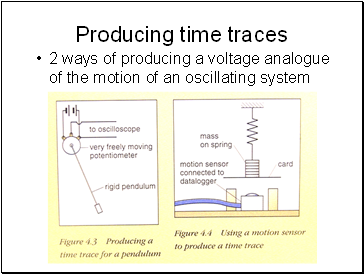## Producing time traces

2 ways of producing a voltage analogue of the motion of an oscillating system

Slide 21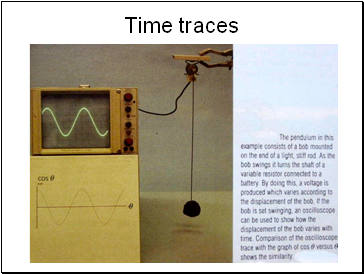Go to page:
1  2  3